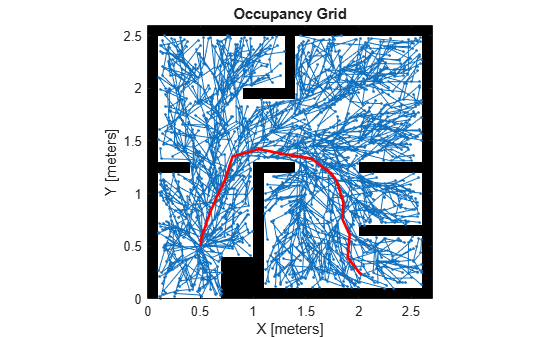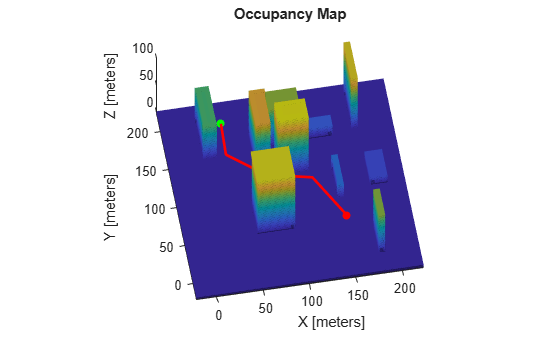# plannerRRTStar

Create an optimal RRT path planner (RRT*)

## Description

The `plannerRRTStar` object creates an asymptotically-optimal RRT planner, RRT*. The RRT* algorithm converges to an optimal solution in terms of the state space distance. Also, its runtime is a constant factor of the runtime of the RRT algorithm. RRT* is used to solve geometric planning problems. A geometric planning problem requires that any two random states drawn from the state space can be connected.

## Creation

### Syntax

``planner = plannerRRTStar(stateSpace,stateVal)``
``planner = plannerRRTStar(___,Name=Value)``

### Description

````planner = plannerRRTStar(stateSpace,stateVal)` creates an RRT* planner from a state space object, `stateSpace`, and a state validator object, `stateVal`. The state space of `stateVal` must be the same as `stateSpace`. `stateSpace` and `stateVal` also sets the StateSpace and StateValidator properties of the `planner` object.```

example

````planner = plannerRRTStar(___,Name=Value)` sets properties using one or more name-value arguments in addition to the input arguments in the previous syntax. You can specify the BallRadiusConstant, ContinueAfterGoalReached, MaxNumTreeNodes, MaxIterations, MaxConnectionDistance, GoalReachedFcn, and GoalBias properties as name-value arguments.```

## Properties

expand all

State space for the planner, specified as a state space object. You can use state space objects such as `stateSpaceSE2`, `stateSpaceDubins`, `stateSpaceReedsShepp`, and `stateSpaceSE3`. You can also customize a state space object using the `nav.StateSpace` object.

State validator for the planner, specified as a state validator object. You can use state validator objects such as `validatorOccupancyMap`, `validatorVehicleCostmap`, and `validatorOccupancyMap3D`.

Constant used to estimate the near neighbors search radius, specified as a positive scalar. With a larger ball radius, the searching radius reduces slower as the number of nodes in the tree increases.

Example: `BallRadiusConstant=80`

Data Types: `single` | `double`

Decide if the planner continues to optimize after the goal is reached, specified as `false` or `true`. The planner also terminates regardless of the value of this property if the maximum number of iterations or maximum number of tree nodes is reached.

Example: `ContinueAfterGoalReached=true`

Data Types: `logical`

Maximum number of nodes in the search tree (excluding the root node), specified as a positive integer.

Example: `MaxNumTreeNodes=2500`

Data Types: `single` | `double`

Maximum number of iterations, specified as a positive integer.

Example: `MaxIterations=2500`

Data Types: `single` | `double`

Maximum length of a motion allowed in the tree, specified as a scalar.

Example: `MaxConnectionDistance=0.3`

Data Types: `single` | `double`

Callback function to determine whether the goal is reached, specified as a function handle. You can create your own goal reached function. The function must follow this syntax:

` function isReached = myGoalReachedFcn(planner,currentState,goalState)`

where:

• `planner` — The created planner object, specified as `plannerRRTStar` object.

• `currentState` — The current state, specified as a three element real vector.

• `goalState` — The goal state, specified as a three element real vector.

• `isReached` — A boolean variable to indicate whether the current state has reached the goal state, returned as `true` or `false`.

To use custom `GoalReachedFcn` in code generation workflow, this property must be set to a custom function handle before calling the plan function and it cannot be changed after initialization.

Data Types: `function handle`

Probability of choosing the goal state during state sampling, specified as a real scalar in range [0,1]. The property defines the probability of choosing the actual goal state during the process of randomly selecting states from the state space. You can start by setting the probability to a small value such as `0.05`.

Example: `GoalBias=0.1`

Data Types: `single` | `double`

## Object Functions

 `plan` Plan path between two states `copy` Create copy of planner object

## Examples

collapse all

Create a state space.

`ss = stateSpaceSE2;`

Create an `occupancyMap`-based state validator using the created state space.

`sv = validatorOccupancyMap(ss);`

Create an occupancy map from an example map and set map resolution as 10 cells/meter.

```load exampleMaps.mat map = occupancyMap(simpleMap,10); sv.Map = map;```

Set validation distance for the validator.

`sv.ValidationDistance = 0.01;`

Update state space bounds to be the same as map limits.

`ss.StateBounds = [map.XWorldLimits; map.YWorldLimits; [-pi pi]];`

Create RRT* path planner and allow further optimization after goal is reached. Reduce the maximum iterations and increase the maximum connection distance.

```planner = plannerRRTStar(ss,sv, ... ContinueAfterGoalReached=true, ... MaxIterations=2500, ... MaxConnectionDistance=0.3);```

Set the start and goal states.

```start = [0.5 0.5 0]; goal = [2.5 0.2 0];```

Plan a path with default settings.

```rng(100,'twister') % repeatable result [pthObj,solnInfo] = plan(planner,start,goal);```

Visualize the results.

```map.show hold on % Tree expansion plot(solnInfo.TreeData(:,1),solnInfo.TreeData(:,2),'.-') % Draw path plot(pthObj.States(:,1),pthObj.States(:,2),'r-','LineWidth',2)```Load a 3-D occupancy map of a city block into the workspace. Specify the threshold to consider cells as obstacle-free.

```mapData = load("dMapCityBlock.mat"); omap = mapData.omap; omap.FreeThreshold = 0.5;```

Inflate the occupancy map to add a buffer zone for safe operation around the obstacles.

`inflate(omap,1)`

Create an SE(3) state space object with bounds for state variables.

`ss = stateSpaceSE3([0 220;0 220;0 100;inf inf;inf inf;inf inf;inf inf]);`

Create a 3-D occupancy map state validator using the created state space. Assign the occupancy map to the state validator object. Specify the sampling distance interval.

```sv = validatorOccupancyMap3D(ss, ... Map = omap, ... ValidationDistance = 0.1);```

Create a RRT star path planner with increased maximum connection distance and reduced maximum number of iterations. Specify a custom goal function that determines that a path reaches the goal if the Euclidean distance to the target is below a threshold of 1 meter.

```planner = plannerRRTStar(ss,sv, ... MaxConnectionDistance = 50, ... MaxIterations = 1000, ... GoalReachedFcn = @(~,s,g)(norm(s(1:3)-g(1:3))<1), ... GoalBias = 0.1);```

Specify start and goal poses.

```start = [40 180 25 0.7 0.2 0 0.1]; goal = [150 33 35 0.3 0 0.1 0.6];```

Configure the random number generator for repeatable result.

`rng(1,"twister");`

Plan the path.

`[pthObj,solnInfo] = plan(planner,start,goal);`

Visualize the planned path.

```show(omap) axis equal view([-10 55]) hold on % Start state scatter3(start(1,1),start(1,2),start(1,3),"g","filled") % Goal state scatter3(goal(1,1),goal(1,2),goal(1,3),"r","filled") % Path plot3(pthObj.States(:,1),pthObj.States(:,2),pthObj.States(:,3), ... "r-",LineWidth=2)```Karaman, S. and E. Frazzoli. "Sampling-Based Algorithms for Optimal Motion Planning." The International Journal of Robotics Research. Vol. 30, Number 7, 2011, pp 846 – 894.